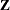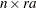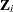Processing by Subjects

Some mixed models can be expressed in different but mathematically equivalent ways with PROC GLIMMIX statements. While equivalent statements lead to equivalent statistical models, the data processing and estimation phase can be quite different, depending on how you write the GLIMMIX statements. For example, the particular use of the SUBJECT= option in the RANDOM statement affects data processing and estimation. Certain options are available only when the data are processed by subject, such as the EMPIRICAL option in the PROC GLIMMIX statement.

Consider a GLIMMIX model where variables A and Rep are classification variables withandlevels, respectively. The following pairs of statements produce the same random-effects structure:

class Rep A;
random Rep*A;
class Rep A;
random intercept / subject=Rep*A;
class Rep A;
random Rep / subject=A;
class Rep A;
random A / subject=Rep;

In the first case, PROC GLIMMIX does not process the data by subjects because no SUBJECT= option was given. The computation of empirical covariance estimators, for example, will not be possible. The marginal variance-covariance matrix has the same block-diagonal structure as for cases 2–4, where each block consists of the observations belonging to a unique combination of Rep and A. More importantly, the dimension of thematrix of this model will be, andwill be sparse. In the second case, thematrix for each of thesubjects is a vector of ones.

If the data can be processed by subjects, the procedure typically executes faster and requires less memory. The differences can be substantial, especially if the number of subjects is large. Recall that fitting of generalized linear mixed models might be doubly iterative. Small gains in efficiency for any one optimization can produce large overall savings.

If you interpret the intercept as "1," then a RANDOM statement with TYPE=VC (the default) and no SUBJECT= option can be converted into a statement with subject by dividing the random effect by the eventual subject effect. However, the presence of the SUBJECT= option does not imply processing by subject. If a RANDOM statement does not have a SUBJECT= effect, processing by subjects is not possible unless the random effect is a pure R-side overdispersion effect. In the following example, the data will not be processed by subjects, because the first RANDOM statement specifies a G-side component and does not use a SUBJECT= option:

proc glimmix;
class A B;
model y = B;
random A;
random B / subject=A;
run;

To allow processing by subjects, you can write the equivalent model with the following statements:

proc glimmix;
class A B;
model y = B;
random int / subject=A;
random B   / subject=A;
run;

If you denote a variance component effect X with subject effect S as X–(S), then the "calculus of random effects" applied to the first RANDOM statement reads A = Int*A = Int–(A) = A–(Int). For the second statement there are even more equivalent formulations: A*B = A*B*Int = A*B–(Int) = A–(B) = B–(A) = Int–(A*B).

If there are multiple subject effects, processing by subjects is possible if the effects are equal or contained in each other. Note that in the last example the A*B interaction is a random effect. The following statements give an equivalent specification to the previous model:

proc glimmix;
class A B;
model y = B;
random int / subject=A;
random A   / subject=B;
run;

Processing by subjects is not possible in this case, because the two subject effects are not syntactically equal or contained in each other. The following statements depict a case where subject effects are syntactically contained:

proc glimmix;
class A B;
model y = B;
random int / subject=A;
random int / subject=A*B;
run;

The A main effect is contained in the A*B interaction. The GLIMMIX procedure chooses as the subject effect for processing the effect that is contained in all other subject effects. In this case, the subjects are defined by the levels of A.

You can examine the "Model Information" and "Dimensions" tables to see whether the GLIMMIX procedure processes the data by subjects and which effect is used to define subjects. The "Model Information" table displays whether the marginal variance matrix is diagonal (GLM models), blocked, or not blocked. The "Dimensions" table tells you how many subjects (=blocks) there are.

Finally, nesting and crossing of interaction effects in subject effects are equivalent. The following two RANDOM statements are equivalent:

class Rep A;
random intercept / subject=Rep*A;
class Rep A;
random intercept / subject=Rep(A);